Question

# A unity feedback control system has the open loop TF as

A unity feedback control system has the open loop TF as: $$G(s)=\frac{K(s+a+1)(s+b)}{s(s+a)(s+a+2)}$$

a) Find analytical expressions for the magnitude and phase response for $$\mathrm{G}(\mathrm{s}) .\left[K=K_{1}\right]$$

b) Make a plot of the log-magnitude and the phase, using log-frequency in rad/s as the ordinate. $$\left[K=K_{1}\right]$$

c) Sketch the Bode asymptotic magnitude and asymptotic phase plots. $$\left[K=K_{1}\right]$$

d) Compare the results from $$(a),(b)$$, and $$(c) .\left[K=K_{1}\right]$$

e) Using the Nyquist criterion, find out if system is stable. Show your steps. $$\left[K=K_{1}\right]$$

f) Using the Nyquist criterion, find the range of $$K$$ for stability.

g) Use bode plots to calculate the gain and phase margins if the value of $$K$$ is:

- $$0.1 K_{1}$$

- $$10 K_{1}$$

- $$100 K_{1}$$

h) Use Matlab to check the results from (g).

i) Use Matlab to display the gain and phase margins on:

- Nyquist plot

- Bode plot

We need at least 10 more requests to produce the answer.

0 / 10 have requested this problem solution

The more requests, the faster the answer.

All students who have requested the answer will be notified once they are available.

#### Earn Coins

Coins can be redeemed for fabulous gifts.

Similar Homework Help Questions
• ### Bode Plots Sketch the Bode plot magnitude and phase for each of the three open-loop transfer...

Bode Plots Sketch the Bode plot magnitude and phase for each of the three open-loop transfer functions listed below. Verify your results using the bode m function in MATLAB.(a) $$G(s)=\frac{100}{s(0.1 s+1)(0.01 s+1)}$$(b) $$G(s)=\frac{1}{(s+1)^{2}\left(s^{2}+s+9\right)}$$(c) $$G(s)=\frac{16000 s}{(s+1)(s+100)\left(s^{2}+5 s+1600\right)}$$

• ### A unity feedback system has the following open-loop gain function 10 s(s+2) Use MATLAB to plot...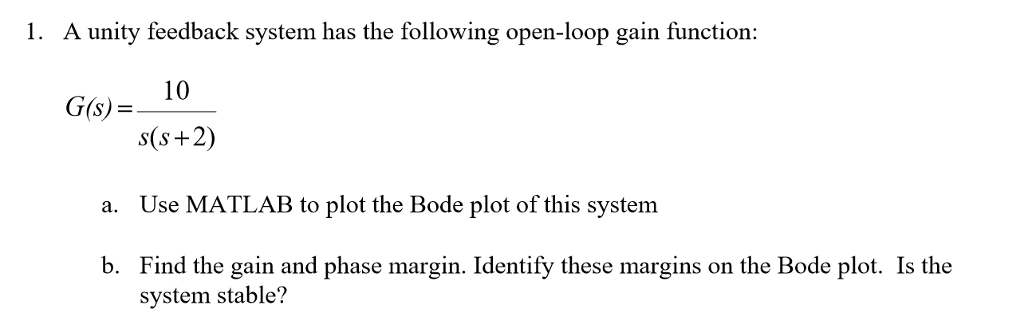A unity feedback system has the following open-loop gain function 10 s(s+2) Use MATLAB to plot the Bode plot of this system Find the gain and phase margin. Identify these margins on the Bode plot. Is the G(s) a. b. system stable?

• ### For the unity feedback system in the below figure, 1. EGO) R(s)) C(s) G(s)K (s 1) (s + 4) a) Sket...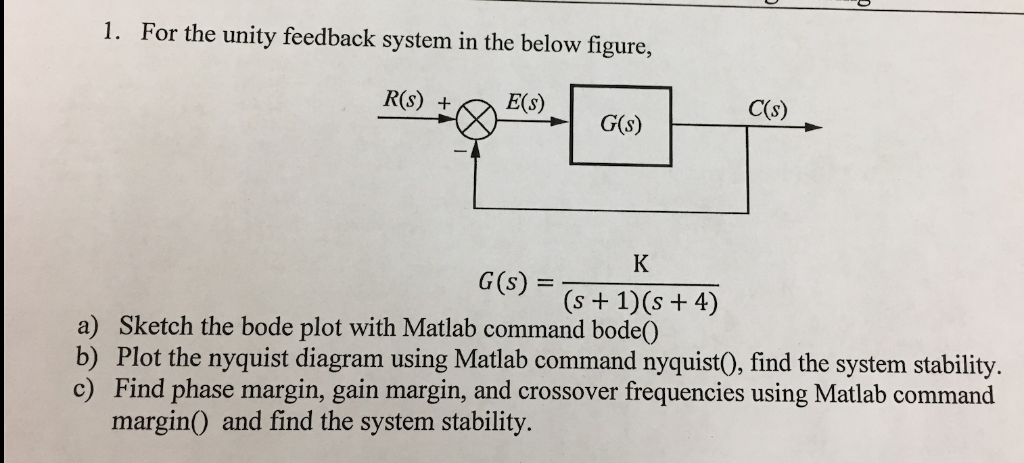For the unity feedback system in the below figure, 1. EGO) R(s)) C(s) G(s)K (s 1) (s + 4) a) Sketch the bode plot with Matlab command bode0 b) Plot the nyquist diagram using Matlab command nyquist(0, find the system stability c) Find phase margin, gain margin, and crossover frequencies using Matlab command margin(0 and find the system stability For the unity feedback system in the below figure, 1. EGO) R(s)) C(s) G(s)K (s 1) (s + 4) a) Sketch...

• ### 1) (10 pts) Consider the unity feedback system shown in the figure: For each of the...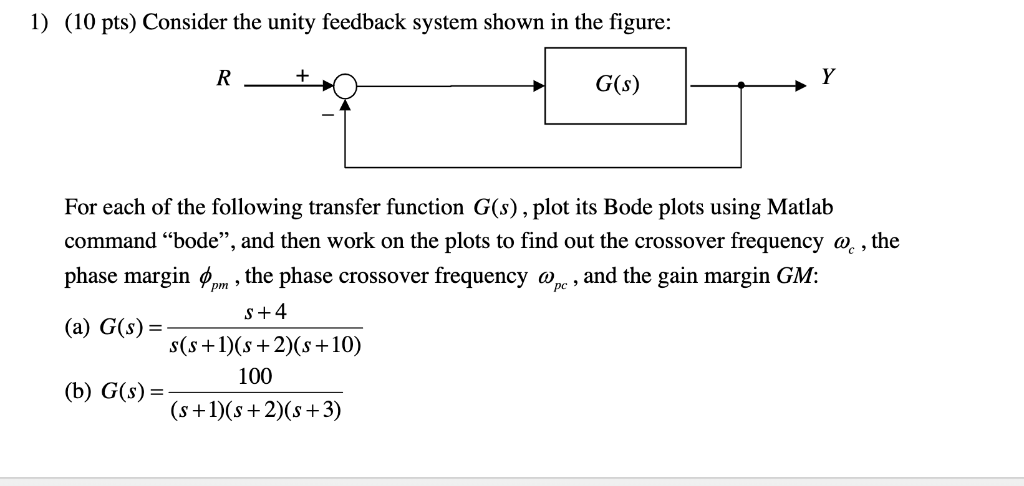1) (10 pts) Consider the unity feedback system shown in the figure: For each of the following transfer function G(s), plot its Bode plots using Matlab command "bode", and then work on the plots to find out the crossover frequency phase margin . the phase crossover frequency and the gain margin GM: (a) G(s)= , the S+4 s(s + l)(s + 2)(s +10) (b) Gs)100

• ### A unity gain negative feedback system has an open-loop transfer function given by 4. s) =...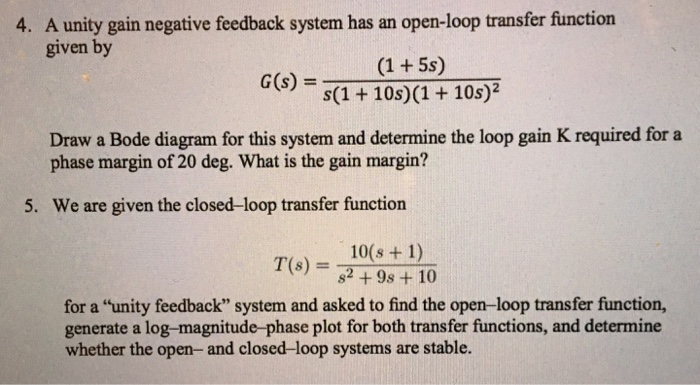A unity gain negative feedback system has an open-loop transfer function given by 4. s) = s(1 + 10s)(1 + 10s)? Draw a Bode diagram for this system and determine the loop gain K required for a phase margin of 20 deg. What is the gain margin? 5. We are given the closed-loop transfer function 10(s + 1) T(s) = 82+98+10 for a "unity feedback" system and asked to find the open-loop transfer function, generate a log-magnitude-phase plot for both...

• ### a=8 Q.17,3,3,3, 2, 1, 1] Consider the unity feedback system: 10 (5) (Where "a" is the...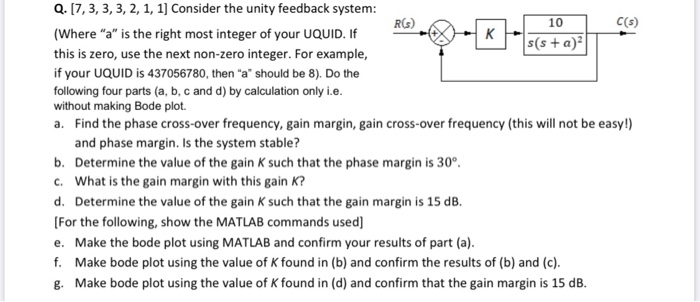a=8 Q.17,3,3,3, 2, 1, 1] Consider the unity feedback system: 10 (5) (Where "a" is the right most integer of your UQUID. If Ss(s+a) | this is zero, use the next non-zero integer. For example, if your UQUID is 437056780, then "a" should be 8). Do the following four parts (a, b, c and d) by calculation only i.e. without making Bode plot. a. Find the phase cross-over frequency, gain margin, gain cross-over frequency (this will not be easy!) and...

• ### 3. Construct the bode plot on a semilog Graph-paper for a unity feedback system whose open...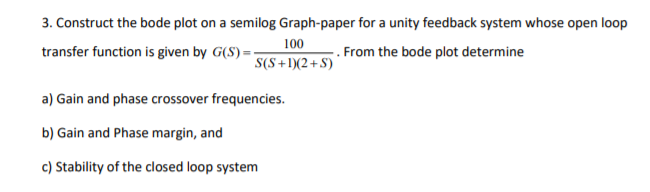Construct the bode plot on a semilog Graph-paper for a unity feedback system whose open looptransfer function is given by $$G(S)=\frac{100}{S(S+1)(2+S)} .$$ From the bode plot determinea) Gain and phase crossover frequencies.b) Gain and Phase margin, andc) Stability of the closed loop system

• ### For the given system (s+25) P(s) s(s+1)(s+3000) 1. Sketch, by hand, the Bode asymptote plots for phase and magnitude. S...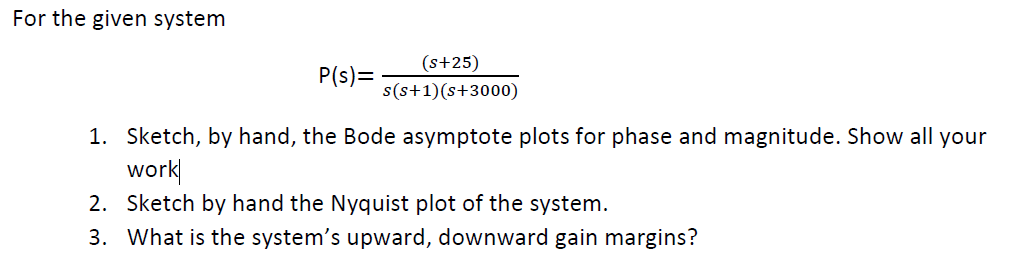For the given system (s+25) P(s) s(s+1)(s+3000) 1. Sketch, by hand, the Bode asymptote plots for phase and magnitude. Show all your work 2. Sketch by hand the Nyquist plot of the system 3. What is the system's upward, downward gain margins? For the given system (s+25) P(s) s(s+1)(s+3000) 1. Sketch, by hand, the Bode asymptote plots for phase and magnitude. Show all your work 2. Sketch by hand the Nyquist plot of the system 3. What is the system's...

• ### 1. The open loop system G()l be placed into a unity feedback system s2(s+1) as shown below. a. Sk...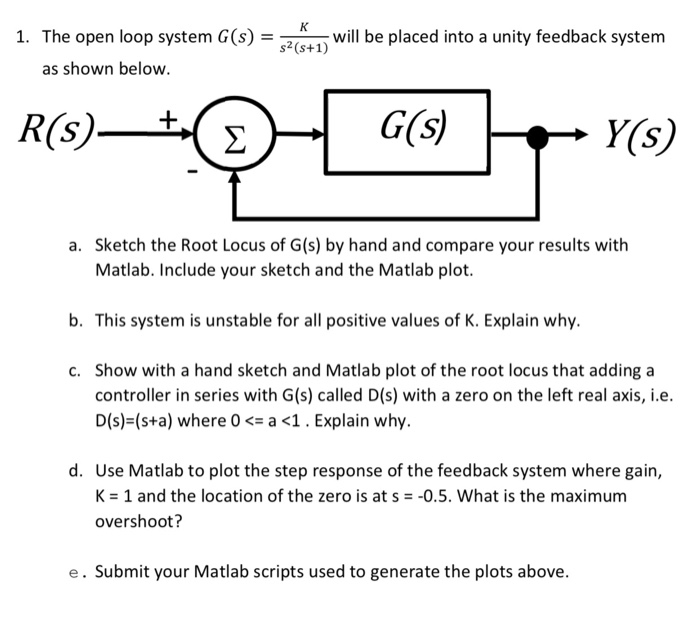please answer all parts and show the related work. thank you! especially the matlab parts! 1. The open loop system G()l be placed into a unity feedback system s2(s+1) as shown below. a. Sketch the Root Locus of G(s) by hand and compare your results with Matlab. Include your sketch and the Matlab plot. b. This system is unstable for all positive values of K. Explain why. c. Show with a hand sketch and Matlab plot of the root locus...